# 【问题解答】Python中非十进制数做参数会自动转换成十进制么？

Python 3289浏览

Python中非十进制数做参数会自动转换成十进制么？-CSDN论坛

```比如chr()函数，文档中说参数i应该是integer，但是输入0o47055、0x4e2d、0b100111000101101都可以得到字符“中”。

【问题1】比如chr()函数，文档中说参数i应该是integer，但是输入0o47055、0x4e2d、0b100111000101101都可以得到字符“中”。

10进制 20013

• 10进制的：20013

• 8进制：0o47055
• 16进制：0x4e2d
• 2进制：0b100111000101101

```chr(20013)
chr(0o47055)
chr(0x4e2d)
chr(0b100111000101101)```

-》所以可以回复你的问题了：

（1）不同进制的数字之间如何转换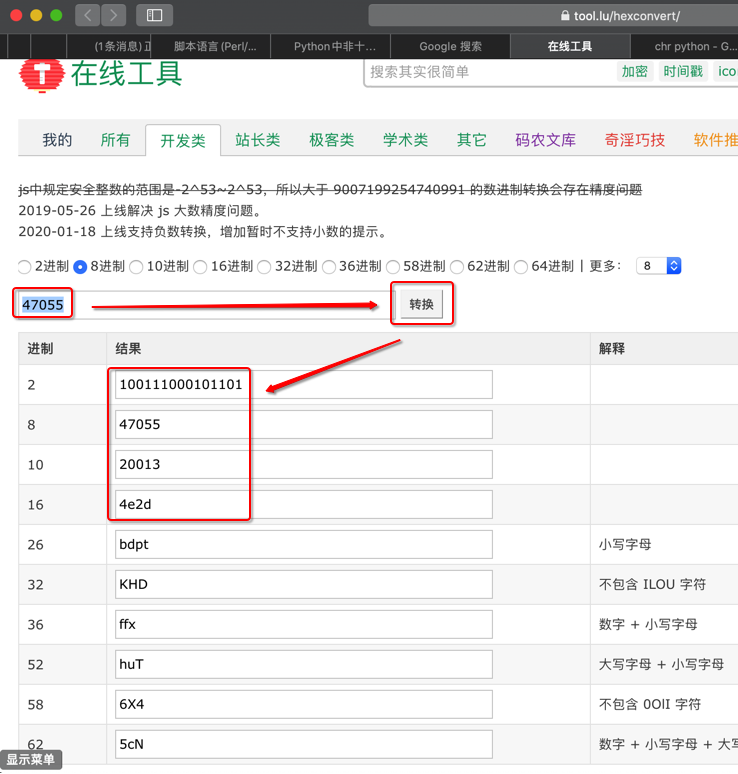（2）关于python中chr

chr python

Python chr() 函数 | 菜鸟教程

Built-in Functions — Python 3.8.1 documentation

“chr(i)

Return the string representing a character whose Unicode code point is the integer i. For example, chr(97) returns the string ‘a’, while chr(8364) returns the string ‘€’. This is the inverse of ord().

The valid range for the argument is from 0 through 1,114,111 (0x10FFFF in base 16).  ValueErrorwill be raised if i is outside that range.”

“chr(i)

chr()，输入的参数是：Unicode的码位，也叫编码值，标准叫法是：unicode code point

（3）此处提到的：中国的”中”字的Unicode的码位

unicode 20013

unicode code point

Unicode codepoint lookup/search toolCharacter: 中          (U+4E2D)

Mac中搜狗输入法中也可以输入的微笑的表情图标：😊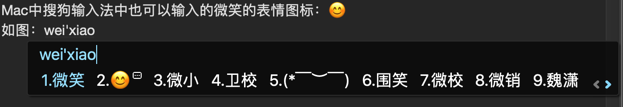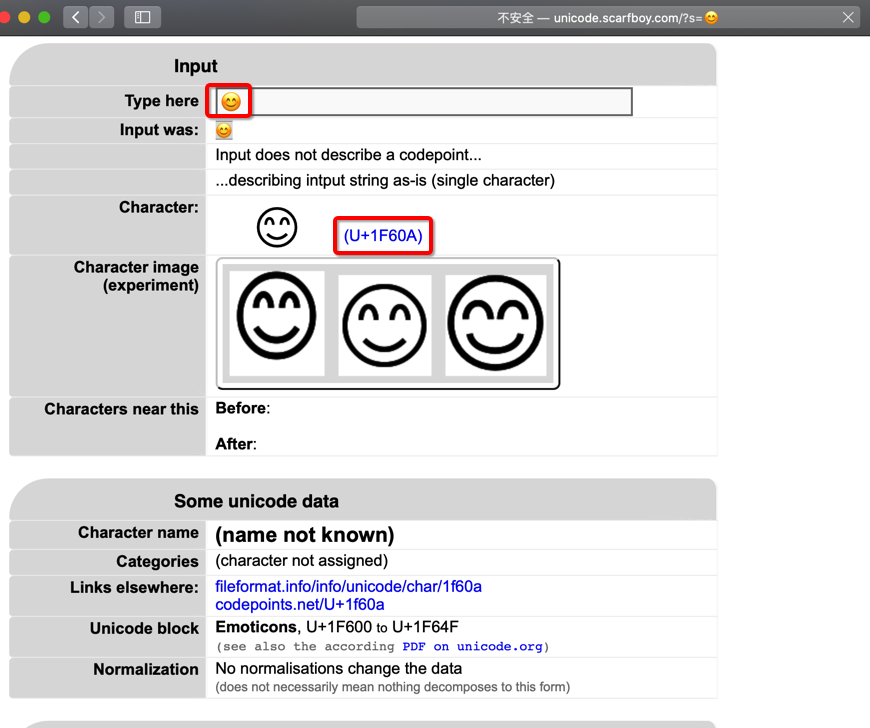【问题2】还有就是网上写的2进制、8进制、16进制互转，用内置函数的话都是用int()中转成10进制，再用bin()、oct()、hex()这几个内置函数转成相应的进制。但是我发现bin()可以直接输入8进制和16进制数，返回2进制数

python int()

Built-in Functions — Python 3.8.1 documentation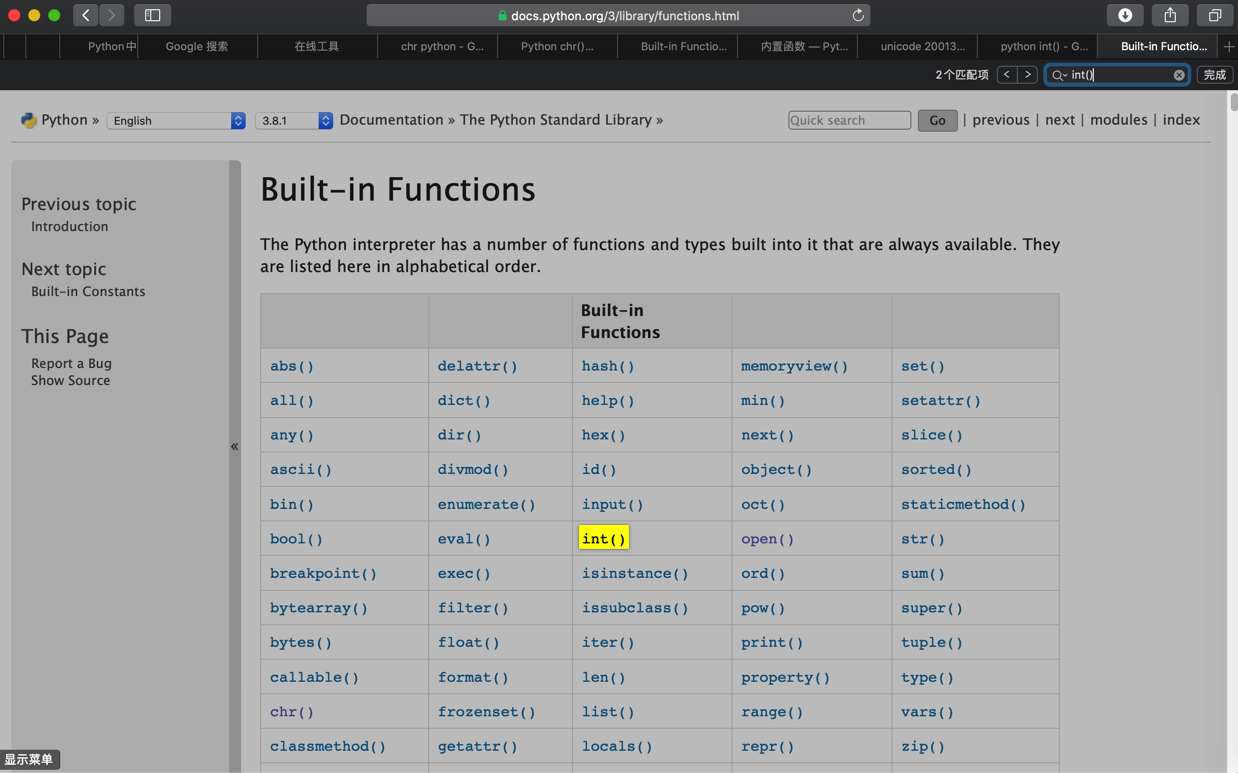class int(x, base=10)

Return an integer object constructed from a number or string x, or return 0 if no arguments are given. If x defines __int__(), int(x) returns x.__int__(). If x defines __index__(), it returns x.__index__(). If x defines __trunc__(), it returns x.__trunc__(). For floating point numbers, this truncates towards zero.

If x is not a number or if base is given, then x must be a string, bytes, or bytearray instance representing an integer literal in radix base. Optionally, the literal can be preceded by + or – (with no space in between) and surrounded by whitespace. A base-n literal consists of the digits 0 to n-1, with a to z (or A to Z) having values 10 to 35. The default base is 10. The allowed values are 0 and 2–36. Base-2, -8, and -16 literals can be optionally prefixed with 0b/0B, 0o/0O, or 0x/0X, as with integer literals in code. Base 0 means to interpret exactly as a code literal, so that the actual base is 2, 8, 10, or 16, and so that int(‘010’, 0) is not legal, while int(‘010’) is, as well as int(‘010’, 8).

The integer type is described in Numeric Types — int, float, complex.

Changed in version 3.4: If base is not an instance of int and the base object has abase.__index__ method, that method is called to obtain an integer for the base. Previous versions used base.__int__ instead of base.__index__.

Changed in version 3.6: Grouping digits with underscores as in code literals is allowed.

Changed in version 3.7: x is now a positional-only parameter.

Changed in version 3.8: Falls back to __index__() if __int__() is not defined.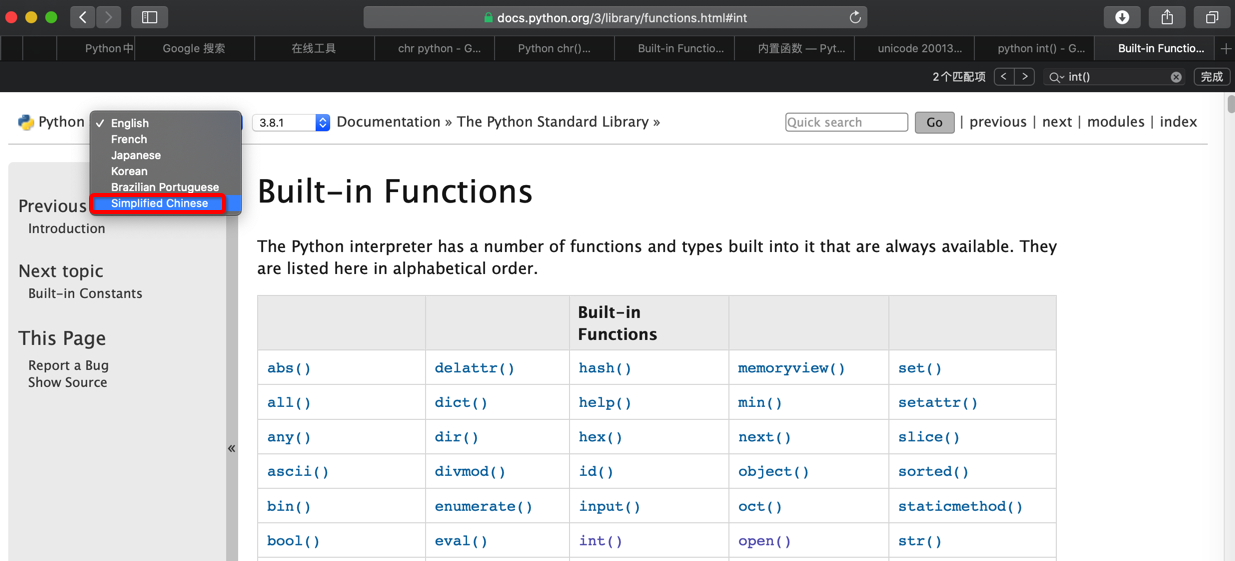-》

https://docs.python.org/zh-cn/3/library/functions.html#int

“class int([x])

class int(x, base=10)

```# Function: demo for
#     Python中非十进制数做参数会自动转换成十进制么？-CSDN论坛
#     https://bbs.csdn.net/topics/395822961
# Author: Crifan Li
# Update: 20200202

intBase10 = 20013
print("intBase10=%s" % intBase10)

intBase10Str = "20013"
convertedInt10FromBase10 = int(intBase10Str)
print("convertedInt10FromBase10=%s" % convertedInt10FromBase10)

intBase2Str = "0b100111000101101"
convertedInt10FromBase2 = int(intBase2Str, base=2)
print("convertedInt10FromBase2=%s" % convertedInt10FromBase2)

intBase8Str = "0o47055"
convertedInt10FromBase8 = int(intBase8Str, base=8)
print("convertedInt10FromBase8=%s" % convertedInt10FromBase8)

intBase16Str = "0x4e2d"
convertedInt10FromBase16 = int(intBase16Str, base=16)
print("convertedInt10FromBase16=%s" % convertedInt10FromBase16)

# intBase10=20013
# convertedInt10FromBase10=20013
# convertedInt10FromBase2=20013
# convertedInt10FromBase8=20013
# convertedInt10FromBase16=20013```

• 2进制：bin()
• 将一个整数转变为一个前缀为“0b”的二进制字符串。结果是一个合法的 Python 表达式。如果 x 不是 Python 的 int 对象，那它需要定义 __index__() 方法返回一个整数。一些例子：
• >>> bin(3)
• ‘0b11’
• >>> bin(-10)
• ‘-0b1010’
• 8进制：oct()
• oct(x)
• 将一个整数转变为一个前缀为“0o”的八进制字符串。结果是一个合法的 Python 表达式。如果 x 不是 Python 的 int 对象，那它需要定义 __index__() 方法返回一个整数。一些例子：
• >>> oct(8)
• ‘0o10’
• >>> oct(-56)
• ‘-0o70’
• 16进制：
• hex()

• 输入：整数 Integer=int
• 比如 3，8，255之类的
• 输出：字符串 string=str
• 对应的该种进制的写法，比如：’0b11’，’0o10’，’0xff’

```binStr = bin(intBase10)
print("binStr=%s" % binStr)

octStr = oct(intBase10)
print("octStr=%s" % octStr)

hexStr = hex(intBase10)
print("hexStr=%s" % hexStr)

# binStr=0b100111000101101
# octStr=0o47055
# hexStr=0x4e2d```

• Python中的基本的内置函数：int(), bin(), oct(), hex()
• 官网文档
• 英文：
• 不同进制的数字的表达方式，写法
• 比如：20013 = 0b100111000101101 = 0o47055 = 0x4e2d
• Unicode的code point
• 属于：字符编码
• 不熟悉的可参考我的教程：
• 详解：

87 queries in 0.109 seconds, using 20.65MB memory